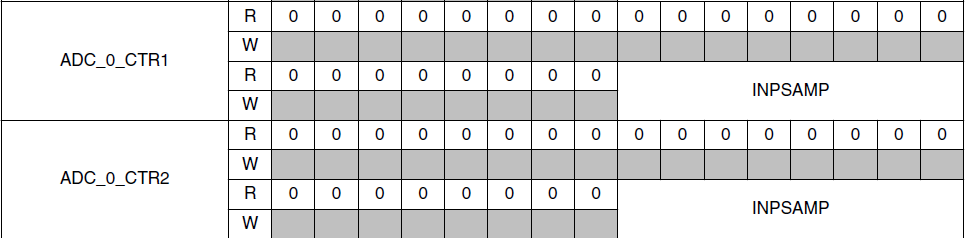## Question regarding ADC Example project in MPC5748G

cancel
Showing results for
Search instead for
Did you mean:
SOLVED

## Question regarding ADC Example project in MPC5748G

Jump to solution
246 ViewsContributor II

I've been running the ADC example code in S32DS, which converts the ADC result to illuminate the LED light on the MPC7548G board. The example code contains ADC1_Calibration(), ADC_Init(), ADC1_Read_Chan() and update_LEDs(), which works fine.

1) My question is how to set the ADC sampling rate? I want to implement the 'oversampling' method into my project but couldn't find where to set. Let's say I want to manually set the ADC sampling rate at 20KHz, and the incoming analog signal is 10KHz.

2) Also the example project only reads and stores the latest value of the ADC conversion result, how to store and monitor the entire ADC conversion result(continuously)? Is there a way to monitor the ADC conversion result in real-time or store it somewhere so I can check it later?

Reference Codes from the Example:

void ADC1_Init(void) { /* Initialize ADC1 module & start normal scan mode */
ADC_1.MCR.B.PWDN = 1; /* Power down for starting module initialization */
ADC_1.MCR.B.OWREN = 1; /* Enable overwriting older conversion results */
ADC_1.MCR.B.MODE = 1; /* Scan mode (1) used instead of one shot mode */
ADC_1.MCR.B.ADCLKSEL = 1; /* ADC clock = FS80 bus clock (80 MHz here) */
ADC_1.MCR.B.PWDN = 0; /* ADC_1 ready to receive conversation triggers */
ADC_1.MCR.B.NSTART = 1; /* Initiate trigger for normal scan */
}

void ADC1_Read_Chan (void) { /* Read conversion results */
Result= ADC_1.CDR.B.CDATA; /* Read channel 9 conversion result data */
ResultInMv = (uint16_t) (ADC_VREF*Result/0xFFF); /* Conversion in mV */
}

void ADC1_Calibration(void) { /* Calibrate to compensate for variations. Steps below are from reference manual */
uint32_t __attribute__ ((unused)) ADC1_Calibration_Failed = 1; /* Calibration has not passed yet */

ADC_1.MCR.B.PWDN = 1; /* Power down for starting calibration process */
ADC_1.MCR.B.ADCLKSEL = 0; /* ADC clock = bus clock/2 (80/2 MHz FS80) */
/* Note: Calibration requires it to be set at max 40MHz for best results */
ADC_1.CALBISTREG.B.TEST_EN = 1; /* Enable calibration test */
ADC_1.MCR.B.PWDN = 0; /* Power back up for calibration test to start */
while(ADC_1.CALBISTREG.B.C_T_BUSY); /* Wait for calibration to finish */
if(ADC_1.MSR.B.CALIBRTD) { /* If calibration ran successfully */
ADC1_Calibration_Failed = 0; /* Calibration was successful */
}
else {
ADC1_Calibration_Failed = 1; /* Calibration was not successful */
}

}

void update_LEDs(void) { /* Update LEDs with scaled channel 9 Result */
SIUL2.GPDO[PA4].R = ~(Result & 0x0800)>>11;/* LED1: scaled channel 9 LSB */
SIUL2.GPDO[PA0].R = ~(Result & 0x0400)>>10;/* LED2 */
SIUL2.GPDO[PJ4].R = ~(Result & 0x0200)>>9; /* LED3 */
SIUL2.GPDO[PH5].R = ~(Result & 0x0100)>>8; /* LED4 */
SIUL2.GPDO[PC4].R = ~(Result & 0x0080)>>7; /* LED5 */
SIUL2.GPDO[PH13].R = ~(Result & 0x0040)>>6;/* LED6 */
SIUL2.GPDO[PA7].R = ~(Result & 0x0020)>>5; /* LED7 */
SIUL2.GPDO[PA10].R = ~(Result & 0x0010)>>4; /* LED8: scaled channel 9 MSB */
}

Labels (1)
• ### General

Tags (2)
1 Solution
140 ViewsNXP TechSupport

Hi,

for Subsequent/continuous conversion you have

1/sample_rate = ADC_clk/(INPSAMP+(13+1)*4+2) => INPSAMP = ADC_clk/sample_rate - 58

INPSAMP is 255 max so you cannot get 10kHz sample rate for ADC_clk you have (80MHz/2), unless you lower bus clock which is not desired. So keep sample rate eg typical 1MHz and trigger ADC with your rate. Either use injected or BCTU triggered conversion.

BR, Petr

3 Replies
140 ViewsNXP TechSupport

Hi,

1) sampling rate is set by programming CTR0 register. See chapter 32.6.12 Conversion time of the RM for more info.

2) ADC is set to scan mode and it reads converted data once conversion is finished. You can store the result into some array, if you want to do some post-processing.

BR, Petr

140 ViewsContributor II

Hi,

Chapter 32.6.12 says " sample time duration is controlled by the INPSAMP[7:0] filed of conversion timing register ADC_CTRx(x=0..2) for different types of channels...The value in the register represents units of cycle of the AD_clk."

How does it express in the code exactly?(when ADC clock = bus clock(80MHz))

Could you provide an example? (e.x. ADC sampling rate =10KHz)Thanks,

Junyi

141 ViewsNXP TechSupport

Hi,

for Subsequent/continuous conversion you have

1/sample_rate = ADC_clk/(INPSAMP+(13+1)*4+2) => INPSAMP = ADC_clk/sample_rate - 58

INPSAMP is 255 max so you cannot get 10kHz sample rate for ADC_clk you have (80MHz/2), unless you lower bus clock which is not desired. So keep sample rate eg typical 1MHz and trigger ADC with your rate. Either use injected or BCTU triggered conversion.

BR, Petr